### 2.3.3.4 Energy Transport Model - Closure at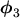and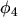By taking the first three moments of BOLTZMANN's transport equation, eqns. (2.99), (2.100), and (2.102), into account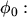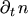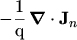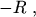(2.147)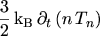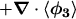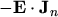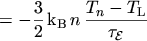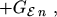(2.148)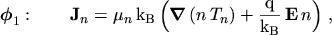(2.149)

an energy transport model is obtained. To close the system the moment of third ordermust be evaluated. This is the only case where we close at an odd moment. For this the anti-symmetric part eqn. (2.111) has to be used. The coefficient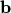found in eqn. (2.111) is determined from the first moment. Since odd moments are calculated, only the anti-symmetric part of the distribution function yields moments different from zero.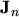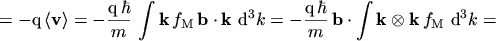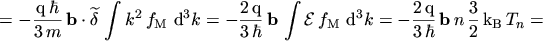(2.150)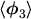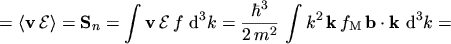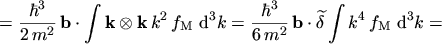(2.151)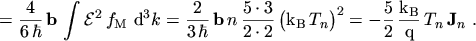Using this closure the 3-moments energy transport model becomes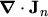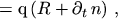(2.152)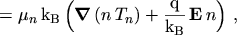(2.153)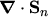(2.154)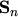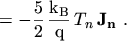(2.155)

The expression for the energy flux density eqn. (2.155) describes pure heat convection. It is often empirically extended by a conductive term where FOURIER's law is used for the heat flow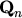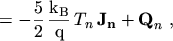(2.156)(2.157)

The thermal conductivity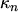is calculated by the WIEDEMANN-FRANZ law, and is proportional to the mobility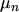and the carrier temperature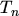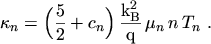(2.158)

Care must be taken to perform this extension in a consistent way.has to be set to zero since the prefactor in eqn. (2.155) reads. In the literature this is often found inconsistent .

However, the heat flow term comes naturally into existence when the first four moment eqns. (2.99), (2.100), (2.102), and (2.103) are taken into account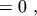(2.159)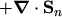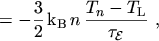(2.160)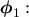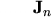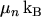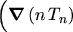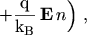(2.161)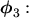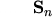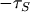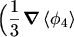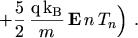(2.162)

Together with the closure relation derived from a heated MAXWELLian, eqn. (2.125),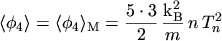(2.163)

the resulting energy transport model reads(2.164)(2.165)(2.166)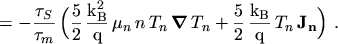(2.167)

In contrast to the drift-diffusion transport model the thermal diffusion current is included in eqn. (2.165) since the gradient operates on both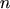and. Moreover, the velocity overshoot effect is included in this equation set sincedepends on, which in turn depends via eqns. (2.166) and (2.167) in a non-local manner on the electric field distribution.

As already mentioned, the heat flow term is present in this model and the WIEDEMANN-FRANZ law foris obtained consistently. Eqn. (2.166) represents the standard form of a conservation equation. The left-hand side represents the energy outflow from some control volume, which must be equal to the sum of the rate of change of the energy density, the energy delivered to the carriers by the electric field per unit volume and time, and the rate of change of energy density due to collisions.

Using an energy transport model, non-local effects like the velocity overshoot are covered. Interestingly, this model also predict a velocity overshoot when the electric field decreases rapidly, for instance at the end of a channel in a MOS transistor. This velocity overshoot is not observed in the more rigorous Monte Carlo simulations and thus termed spurious velocity overshoot. However, it is generally believed that the influence of this effect on device characteristics is small. It appears that the spurious velocity overshoot is a result of the truncation of the moment expansion of BTE at a certain order and close the equation system by some empirical expression. A second point is, that the relaxation times are not single valued functions of the energy. Due to these two reasons it is believed that the spurious velocity overshoot can never be completely eliminated using a finite number of moment equations. More detailed investigations can be found in .

M. Gritsch: Numerical Modeling of Silicon-on-Insulator MOSFETs PDF